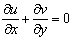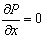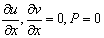﻿ Assessment of Air Flow over an Equilateral Triangular Obstacle in a Horizontal Channel Using FVM

### Assessment of Air Flow over an Equilateral Triangular Obstacle in a Horizontal Channel Using FVMOPEN ACCESSPEER-REVIEWED

## Assessment of Air Flow over an Equilateral Triangular Obstacle in a Horizontal Channel Using FVM

Roya Shademani1, Parviz Ghadimi1,, Rahim Zamanian2, Abbas Dashtimanesh1

1Department of Marine Technology, Amirkabir University of Technology, Tehran, Iran

2International Campus – Mech. Eng’g. Group, Amirkabir University of Technology, Tehran, Iran

### Abstract

Viscous and incompressible flow is simulated over an equilateral triangular obstacle placed in a horizontal channel. Different from the previous studies, the governing Navier-Stokes equations along with appropriate boundary conditions are solved by a particular finite volume generated code. A fixed blockage ratio (β=0.05) is considered. Different ranges of Reynolds numbers in laminar regime under critical value of the Reynolds number (Re ≤ 38.03) are investigated. Range of this study is from 1.4 to 38.03. The flow characteristics are analyzed in the forms of streamlines and pressure fields at various Reynolds numbers. Diagram of drag coefficient versus Reynolds numbers is presented and discussed. Results are compared with the available data in the literature that display good agreements.

### At a glance: Figures

123
Prev Next

• Shademani, Roya, et al. "Assessment of Air Flow over an Equilateral Triangular Obstacle in a Horizontal Channel Using FVM." Journal of Mathematical Sciences and Applications 1.1 (2013): 12-16.
• Shademani, R. , Ghadimi, P. , Zamanian, R. , & Dashtimanesh, A. (2013). Assessment of Air Flow over an Equilateral Triangular Obstacle in a Horizontal Channel Using FVM. Journal of Mathematical Sciences and Applications, 1(1), 12-16.
• Shademani, Roya, Parviz Ghadimi, Rahim Zamanian, and Abbas Dashtimanesh. "Assessment of Air Flow over an Equilateral Triangular Obstacle in a Horizontal Channel Using FVM." Journal of Mathematical Sciences and Applications 1, no. 1 (2013): 12-16.

 Import into BibTeX Import into EndNote Import into RefMan Import into RefWorks

### 1. Introduction

Fluid flow through a horizontal channels is one of the classical problems in the field of fluid mechanics. There are many cases in which some obstacles are in the path of the fluid flows. When separated flow is very large, the obstacle can be classified as bluff bodies. Large number of papers have been published on the study of flow past bluff body cylinders because cylinders like structures are commonly used in the real world and they can be found both in isolation and in groups. Most research in this field is related to circular cylinders. The basis of flow around circular cylinders have been reported in the standard reference by Zdravkovich  where Reynolds numbers range were given for different regimes of flow around smooth circular cylinder in steady flow. The applications and interpretations of data on flow around circular cylinders either experimental data, theoretical models, or computer simulations were comprehensively surveyed by Zdravkovich . Rajani et al  has investigated the wake shapes behind a circular cylinder for both two and three dimensional flow. A comprehensive numerical study for the onset of vortex shedding for flow past variously shaped bodies was investigated by Jackson . Also, Aref et al.  have assessed the bifurcations of the streamline pattern for a bluff body where the vortex wake is formed. Triangular cylinders in cross flow have many engineering applications in cooling of electronic components and heat exchangers, offshore structures and flow metering devices, etc.

Abbassi et al.  studied the two dimensional forced convection of air on a triangular prism in a horizontal channel and observed the transition point at Reynolds=45 for a blockage ratio of 0.25. Zielinska and Wesferid  numerically assessed the wake flow behind an equilateral triangular obstacle for a fixed blockage ratio of 1/15 in Reynolds number range 34-50. They reported a critical Reynolds of 38.3 for this blockage ratio. De and Dalal  have investigated critical Reynolds = 39.9 for an equilateral triangular cylinder with β=1/15. Based on the obtained results, they have concluded that ﬂow is similar to the unconﬁned ﬂow and is more prone to wake instability. Fluid flow and heat transfer across a long equilateral triangular cylinder was studied by Srikanth et al. . Governing equations were solved by using a commercial CFD solver Fluent (6.3). Zeitoun et al.  have studied air flow for 2-D laminar forced convection heat transfer around horizontal triangular for two configurations vortex and base of triangular facing flow. Chatterjee and Mondal  considered Reynolds range of 50 to 250 with three different values of Prandtl number.

Although many papers have been published on flow around different cylinders, but the lack of wide focus about triangular cylinders in comparison to circular or square cylinders is quite evident. Hence, in the present study, to fill the void mentioned above, flow across an equilateral triangular cylinder for different Reynolds is investigated. Streamlines are analyzed and forces acting on a triangular cylinder in the form of drag coefficients are calculated.

### 2. Geometrical Arrangement And Mathematical Equations

The 2-D confined flow of an incompressible laminar viscous fluid in a channel with an equilateral triangular cylinder is investigated. The geometry of the problem and the assigned coordinate system is shown in Figure 1. Triangular obstacle is located in the middle of the channel and at an upstream distance of Xu=12b from the inlet and at a downstream distance of Xd =20b from the outlet. These values are chosen to reduce the effects of boundaries and to satisfy the free stream condition, and are consistent with other studies in the literature . The ratio of the vertical line of the obstacle to the height of channel is β. To examine the effect of the boundaries near the obstacle on the characteristics of the flow, β=0.05 has been reported as a good ratio from the literature [13, 14].

2.1. Governing Equations

Unsteady, incompressible, laminar Navier-Stokes equations with negligible dissipation effect can be expressed in following forms:

1. Continuity:(1)

2. Momentum(2)

where u, v are the velocity components along x, y directions of a Cartesian coordinate system, respectively, and P is the pressure.

Reynolds number is defined as Re=ρUb/μ. ρ is density of fluid, U is uniform velocity of fluid and μ is fluid viscosity and b is the vertical side of triangular cylinder.

2.2. Boundary Conditions

Boundary Conditions considered are as follows:

• At the inlet boundary Ux=U (m/s), Uy=0,.

• On upper and lower boundary Ux= Uy=0.

• On the surface of the triangular cylinder Ux= Uy=0.

At the exit boundary, the.

### 3. Solution Method

Numerical simulation is performed by using Finite volume based Finite element method to solve the governing partial differential equations in a collocated grid system. Implicit solution for a triangular obstacle with unit thickness in steady state flow is considered. The 2-D incompressible laminar viscous model is selected to account for the low Reynolds number flow consideration. The particular FVM scheme implemented in this study is used perhaps for the first time on a triangular obstacle. Mentioned distinction is related to the replacement of velocities in the continuity equation. Checkerboard problem is a routine difficulty in collocated grid structure. Rie and Chow  have presented an algorithm for solving this sort of problem. Different researchers have used this algorithm in different ways. Karimian and Schneider  created the derivatives of pressure from momentum equations and substituted in the continuity equation. As a result, the velocities in the momentum and continuity equations are different. A second order upwind scheme is used to discretize the convection terms and a central difference scheme is used for the diffusive terms of the momentum equation. Coupled equations for mass and momentum are solved in a steady transient fluid Flow. The convergence criterion for the iteration step is 10-5 and for Time step is 10-6.

A non-uniform structured grid distribution is used. Fine mesh is generated around the triangular obstacle for obtaining high accurate vortex model. All the computations were carried out in an Intel core Dou CPU (3.0 GHz) computer.

### 4. Result and Discussion

Air (Density=1.2Kg/m3) is considered as the working fluid for the present study. Simulation are carried out for Re=50-250 in steady state. Moreover, the results are in instantaneous time with time step 0.1 at the dynamic state until 10, 20, 30 time iterations.

4.1. Validation

In order to validate the generated code, a famous case of a fluid flow problem is investigated. Plane poiseuille is solved by the present code and the obtained velocity profile is compared against analytical results . Figure 2 shows the results for the case Re=100. h is the distance between top and bottom planes and U(y) is the horizontal velocity which is a function of the distance between them.

On the other hand, another good case of numerical verification is the result of fluent simulation. Zeitoun et al.  and De et al.  have calculated drag coefficients for a triangular cylinders placed in a channel. Figure 3 shows the comparison of numerical results with the available data in the literature. Reynolds numbers of 1.4 to 38.03 with blockage ratio of β=0.05 are considered in these simulations. The drag coefficient is computed by(3)

CD is the drag coefficient and FD is the drag force acting on the cylinder surface.

As evidenced in the figure, drag coefficient decreases as Reynolds number increases up from the critical Reynolds, where the flow is still steady. But after that, it increases, slightly.

Figure 2 and Figure 3 display good agreements between the obtained results and the bases of comparison which testify to the accuracy of the developed code. Next, the analysis of flow over an equilateral triangular obstacle in a channel is presented.

4.2. Flow Field

Numerical solutions for flow of air around a triangular obstacle are obtained. Several Reynolds numbers in the range 1.4≤ Re ≤ 38.03 corresponding to air following over a triangular cylinders, are investigated. The selected domain and blockage ratio of 0.05 are kept fixed for the present simulations. At the small Reynolds numbers such as Re=1.4, the air stream flows smoothly past the triangular obstacle with no separation. Boundary layer thickness is very thin and no wake is formed. Streamlines are symmetric like in creeping motion. Figure 4 shows the obtained results. Figure 5 illustrates the flow at another Reynolds number of Re=20.8, which again shows that the air stream flows smoothly past the inclined surfaces while two symmetric eddies are formed behind the obstacle. These symmetric vortices grow up with increasing Reynolds numbers and due to unsteady flows with asymmetric vortices where Reynolds number is greater than 38.03 and streamlines have lost symmetry about the centerline as will be seen later. Separation points are near the end of the inclined surfaces where the boundary element layer is very thin. As shown in Figure 6, there are symmetric streamlines around the equilateral triangular cylinder in the case of laminar flow at low Reynolds number. The asymmetry is significant with Reynolds number increasing and some references  and  state that the critical Reynolds number is between Re=38 and Re=38.5. Furthermore, Figure 7 is constructed to obtain the center of eddies l as a function of the Reynolds numbers where l is defined as the distance of eddy’s center from the inlet. It is clear from the figure that, these points are before the instabilities take place and the start of asymmetry flow. Increasing the Reynolds increases eddy’s center move away from triangular vertical side.

### 5. Conclusions

Laminar viscous flow over a triangular cylinder with far boundary channel is investigated by a particular Finite volume scheme. Different ranges of Reynolds numbers in laminar regime under critical value of the Reynolds number (Re ≤ 38.03) are investigated. Velocity vectors show that, for very low Reynolds number which is between 1.4 up 38.03(Recritical), the flow is symmetric around the cylinder with no separation points for vertex facing the flow. Streamlines and flow parameters like drag coefficient are investigated. With increasing Reynolds number and up to critical Reynolds number, flow is still symmetric but eddies are formed and symmetric flow region decreases and changes to a an asymmetric region. Drag coefficients on equilateral triangular cylinder decrease with increasing Reynolds number in symmetric regions and are in qualitative agreement with corresponding data reported in the literature.

Figure 7. Center of eddies l/b as a function of Reynolds number for steady and symmetry flow

### References

  Zdravkovich, M., M., “Flow around circular cylinders”, fundamentals, vol. 1. Oxford university press, USA, 1977.In article  Zdravkovich, M., M., “Flow around circular cylinders”, Applications, 1st ed., Vol.2. Oxford university press, USA, 2002.In article  Rajani, B., N., Kandasamy, A., Majumdar, S., “Numerical simulation of laminar flow past a circular cylinder”, Appl. Math. Model Vol.33, 2008, 1228-1247.In article CrossRef  Jackson, C., P., “A finite volume study of the onset of vortex shedding in flow past variously shaped bodies”, J Fluid Mech., Vol.182, 1987, 23-45.In article CrossRef  Aref, H., Brons, M., Stremler, M., A., “Bifurcation and instability problems in vortex wakes”, J. Comput. Phys. Vol.189, 2003, 351-370.In article  Abbassi, H., Turki, S., Nasrallah, S., “numerical investigation of forced convection in a plane channel with a built-in triangular prism”, J. Thermal Sci, Vol. 40, 2001, 649-658.In article CrossRef  Zielinska, B., J., A., Wesfreid, J., E., “On the spatial structure of global modes in wake flow”, Phys Fluids, Vol.7, 1995, 1418-1424.In article CrossRef  De, A., K., Dalal, A., “Numerical simulation of unconfined flow past a triangular cylinder”, J Numer Meth Fluids, Vol.52, 2006, 801-821.In article CrossRef  De, A., K., Dalal, A., “Numerical study of laminar forced convection ﬂuid ﬂow and heat transfer from a triangular cylinder placed in a channel”, J. Heat Transf, Vol.129, No.5, 2007, 646–656.In article CrossRef  Srikanth, S., Dhiman, A., K., Bijjam, S., “Confined flow and heat transfer across a triangular cylinder in a channel”, J. Thermal Sci, Vol.49, 2011, 2191-2200.In article  Zeitoun, O., Mohamed, A., Nuhait, A., “convective heat transfer around a triangular cylinder in an air cross flow”, J. thermal sciences, Vol. 50, 2011, 1685-1697.In article CrossRef  Chatterjee, D., Mondal, B., “Forced convection heat transfer from an equilateral triangular cylinder at low Reynolds numbers”, Heat mass Trans., Vol. 48, 2012, 1575-1587.In article  Coutanceau, M., Bouard, R., “Experimental determination of the main features of the viscous flow in the wake of a circular cylinder in uniform translation”, Part 1, Steady flow. J Fluid Mech, Vol.79, 1977, 231-265.In article CrossRef  Sohankar, A., Norbeg, C., Davidson, L., “Low-Reynolds flow around a square cylinder at incidence: study of blockage, onset of vortex shedding and outlet boundary condition”, J. Numer. Meth. Fluids, Vol. 26, 1998, 39-56.In article CrossRef  Rhie, C., M., Chow, W., L., “Numerical study of the turbulent flow past an airfoil with trailing edge separation”, AIAA Journal, Vol. 21, 1983, 1525-1532.In article CrossRef  Karimian, S., M., H., Schneider, G., E., “Pressure-based Computational Method for Compressible and Incompressible Flows”, J. Thermo phy. & heat Trans., Vol. 8, No.2, 1994, 267-274.In article  White Frank M., “Viscous fluid flow”, Third edition, International edition 2006, McGraw-Hill publication.In article